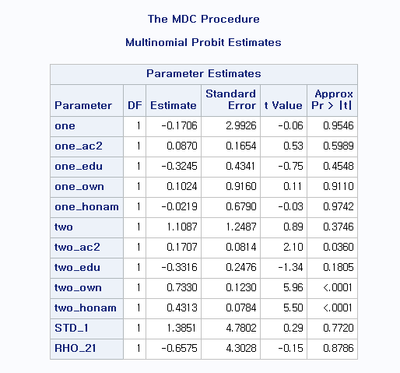## Proc MDC "At least one element of the gradient is greater than 1e-3"

Hi,

I am running multinomial probit model using proc mdc. It gives me the note: At least one element of the gradient is greater than 1e-3.  Can anybody help me how to solve this problem?

This is the code.

proc mdc data=b2015;
model decision=one one_ac2 one_edu one_own one_honam
two two_ac2 two_edu two_own two_honam
/type=mprobit
nchoice=3;
id subject;
run;

The dependent  variable denotes the number of birth(0, 1, or ge than 2). The reference group is 0 birth. When I use 'clogit' instead of 'mprobit' for type, it gives the exact same results with the multinomial logit model. And there is no note. So I think there is no problem within the data. I used diverse options but I could not solve the problem. I hope someone can help me.

Thanks.

Kyungjae

6 REPLIES 6

## Re: Proc MDC "At least one element of the gradient is greater than 1e-3"

Are any of these classification variables? If so, add them to a CLASS statement.

The procedure tries to find parameter estimates that maximize the likelihood of the model, given the data. At the optimal parameter values, the gradient of the likelihood function will be zero. The message is telling you that the procedure was not able to find an optimal set of parameters, but stopped due to some other criterion. You can read about the nonlinear optimization method that PROC MDC uses. You can use the ITPRINT option on the MODEL statement to monitor convergence.

There are many reasons for not finding an optimum. One is a poor choice for the initial guess for the parameters. You can use the INITIAL= option on the MODEL statement to specify an initial guess, or you can use the RANDINT option to generate random initial guesses.

Another possible reason is that the data do not fit the model. Try using a simpler model (fewer explanatory variables, like maybe 5 or 6) to see if you can get convergence with a simpler model.  Then add variables one-by-one to see if you can increase the complexity.

## Re: Proc MDC "At least one element of the gradient is greater than 1e-3"

There is another note that shows up together: Convergence criterion (GCONV=1E-8) satisfied.  I think optimization worked properly.

All the independent variables are dummy variables, so I used Class statement but it does not work well.

Compared to multinomial logit result, the signs of estimates are same but the statistical significance are different. The estimates of multinomial probit model are less significant. I used many nloptions as you recommended but the note "At least one element of the gradient is greater than 1e-3" keeps appearing.

The multinomial probit result is as follows.## Re: Proc MDC "At least one element of the gradient is greater than 1e-3"

> There is another note that shows up together:

Please post the ENTIRE log so that we can see the exact code you submitted and all notes that are displayed when the procedure runs.

## Re: Proc MDC "At least one element of the gradient is greater than 1e-3"

This is the code I run.

proc mdc data=b2015;
model decision=one one_ac2 one_edu one_own one_honam
two two_ac2 two_edu two_own two_honam
/type=mprobit
nchoice=3
id subject;
run;

And these are the notes I get.

NOTE: Convergence criterion (GCONV=1E-8) satisfied.
NOTE: At least one element of the gradient is greater than 1e-3.

Thank you.

## Re: Proc MDC "At least one element of the gradient is greater than 1e-3"

Please post the ENTIRE log so that we can see the exact code you submitted and all notes that are displayed when the procedure runs.

## Re: Proc MDC "At least one element of the gradient is greater than 1e-3"

These are the entire codes I ran. Multinomial Logit model works very well.

libname lkj 'f:/01. korea census/2015_2p';
libname a 'f:/저출산/random coefficient';

data birth2015;
set lkj.h2015;
id=c1;
area=c2*1000+c3;
area1=c2;
if area1=11;
age=c8;
if c8 ge 51 and c8 le 60; *31-40 years old;
if c7=2; *female only;
if c69=99 then c69=0;
if c70=99 then c70=0;
birth=c69+c70; *# of birth;
if birth=. then birth=0;
ac1=0; ac2=0;
if c8 ge 51 and c8 le 55 then ac1=1;
if c8 ge 56 and c8 le 60 then ac2=1;
marry=0;
if c67=2 then marry=1; *married;
mig1=0; mig2=0; mig3=0;
if c19=2 then mig1=1; *non-migrant;
if c19=3 then mig2=1; *migrant from same city;
if c19=4 and area1=c20 then mig2=1; * migrant from same province;
if c19=4 and area1 ne c20 then mig3=1; *migrant from other province;
if c19=5 then delete; *delete migrant from other countries;
if mig1=1 or mig2=1 then mig=0;
if mig3=1 then mig=1;
emp=0;
if c61 in (1 2 3) then emp=1; *employed;
edu=0;
if c13 in (6) and c14=1 then edu=1;
if c13 in ( 7 8 ) then edu=1;*more than bachelor
age_sq=age*age;
if c15=4 then delete; *delete observations who immigrated from other countries;
honam=0;
if c16 in (24 35 36) then honam=1;
htype=0;
if htype2=1 then htype=1; *live in apartment
if own=. then delete;
n_birth=.;
if birth=0 then n_birth=3; *ref.;
if birth=1 then n_birth=1;
if birth ge 2 then n_birth=2;
keep age age_sq area1 area birth ac1 ac2 marry mig1 mig2 mig3 mig own emp edu htype h_age  n_birth c16 honam;
run;

data b2015;
set birth2015;
subject=_n_;
do i=1 to 3;
decision=(n_birth eq i);
one=(i eq 1);
two=(i eq 2);
one_age=one*age;
one_age_sq=one*age_sq;
one_ac1=one*ac1;
one_ac2=one*ac2;
one_edu=one*edu;
one_emp=one*emp;
one_own=one*own;
one_htype=one*htype;
one_h_age=one*h_age;
one_honam=one*honam;
two_age=two*age;
two_age_sq=two*age_sq;
two_ac1=two*ac1;
two_ac2=two*ac2;
two_edu=two*edu;
two_emp=two*emp;
two_own=two*own;
two_htype=two*htype;
two_h_age=two*h_age;
two_honam=two*honam;
output;
end;
run;

/*Multinomial Probit Model*/

proc mdc data=b2015;
model decision=one one_ac2 one_edu one_own one_honam
two two_ac2 two_edu two_own two_honam
/type=mprobit
nchoice=3
maxiter=1000000;
id subject;
nloptions gconv2=0
maxfunc=1000000;
run;

These are all the notes that I got.

NOTE: Convergence criterion (GCONV=1E-8) satisfied.
NOTE: At least one element of the gradient is greater than 1e-3.
NOTE: 프로시저 MDC 실행(총 프로세스 시간):
실행 시간 38.64 초
cpu 시간 38.60 초

Thank you.

Discussion stats
• 6 replies
• 357 views
• 1 like
• 2 in conversation